Like   Tweet
 /* styles */ June 3, 2020 At a time when our grieving nation is finding it difficult to breathe as we mourn George Floyd and too many others, a series of winning Dayton Literary Peace Prize books from the last fourteen years might provide a guide as to the source of the rage and grief, and a path forward to justice. We recommend the following books to you during this complicated time.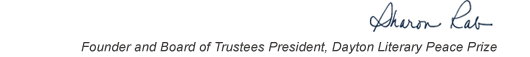table div table+table+table+table div table{width:100%;padding:0}table div table+table+table+table div table img{width:96.23%;padding:0;float:none}table div table+table+table+table div table td{width:100%;padding:0 1.88% 18px}/* styles *//* styles */ We find ourselves in a time when we are confronted with the reality of institutional racism permeating our country. The quote heard lately is, “a riot is the language of the unheard”. And we are encouraged by those in this country and internationally who raise their voices to end injustice. The quality and state of how some move through life is not the norm for marginalized black and brown citizens. The hope is that we recognize our part in dismantling a long history of racism. I am still inspired by the DLPP acceptance speech in 2015 of Bryan Stevenson, Nonfiction winner for “Just Mercy”. I invite you to listen - just listen. Fran Robinson DLPP Communications Chair
 table div table+table+table+table+table+table div table{width:100%;padding:0}table div table+table+table+table+table+table div table img{width:96.23%;padding:0;float:none}table div table+table+table+table+table+table div table td{width:100%;padding:0 1.88% 18px}/* styles *//* styles */ “Read global; buy local.” — Marlon James, DLPP 2009 Fiction Winner for The Book of Night Women
 table div table+table+table+table+table+table+table+table div table{width:100%;padding:0}table div table+table+table+table+table+table+table+table div table img{width:96.23%;padding:0;float:none}table div table+table+table+table+table+table+table+table div table td{width:100%;padding:0 1.88% 18px}/* styles */## Literature, Change, and Peace: The Books

 table div table+table+table+table+table+table+table+table+table+table div table{width:100%;padding:0}table div table+table+table+table+table+table+table+table+table+table div table img{width:96.23%;padding:0;float:none}table div table+table+table+table+table+table+table+table+table+table div table td{width:100%;padding:0 1.88% 18px}/* styles */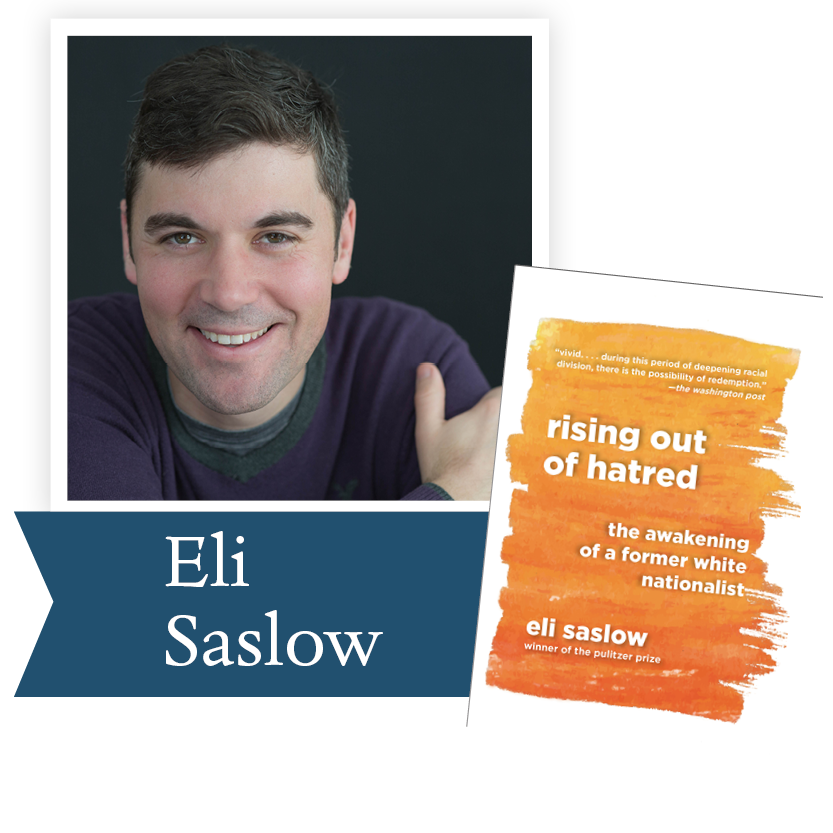2019, Rising Out of Hatred by Eli Saslow, (Doubleday): From a Pulitzer Prize-winning reporter, the powerful story of how a prominent white supremacist changed his heart and mind. With great empathy and narrative verve, Eli Saslow asks what Derek's story can tell us about America's increasingly divided nature. This is a book to help us understand the American moment and to help us better understand one another.

 table div table+table+table+table+table+table+table+table+table+table+table+table div table{width:100%;padding:0}table div table+table+table+table+table+table+table+table+table+table+table+table div table img{width:96.23%;padding:0;float:none}table div table+table+table+table+table+table+table+table+table+table+table+table div table td{width:100%;padding:0 1.88% 18px}/* styles */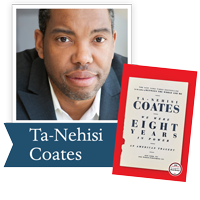2018, We Were Eight Years in Power by Ta-Nehisi Coates (One World PRH): “Biting cultural and political analysis . . . reflects on race, Barack Obama’s presidency and its jarring aftermath, and his evolution as a writer in eight stunningly incisive essays. Kirkus Reviews (starred review)

 table div table+table+table+table+table+table+table+table+table+table+table+table+table+table div table{width:100%;padding:0}table div table+table+table+table+table+table+table+table+table+table+table+table+table+table div table img{width:96.23%;padding:0;float:none}table div table+table+table+table+table+table+table+table+table+table+table+table+table+table div table td{width:100%;padding:0 1.88% 18px}/* styles */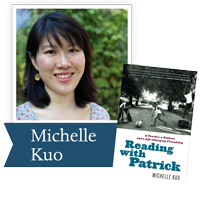2018, Reading with Patrick by Michelle Kuo (Random House): In this stirring memoir, Kuo, the child of Taiwanese immigrants, shares the story of her complicated but rewarding mentorship of one student, Patrick Browning, and his remarkable literary and personal awakening.

 table div table+table+table+table+table+table+table+table+table+table+table+table+table+table+table+table div table{width:100%;padding:0}table div table+table+table+table+table+table+table+table+table+table+table+table+table+table+table+table div table img{width:96.23%;padding:0;float:none}table div table+table+table+table+table+table+table+table+table+table+table+table+table+table+table+table div table td{width:100%;padding:0 1.88% 18px}/* styles */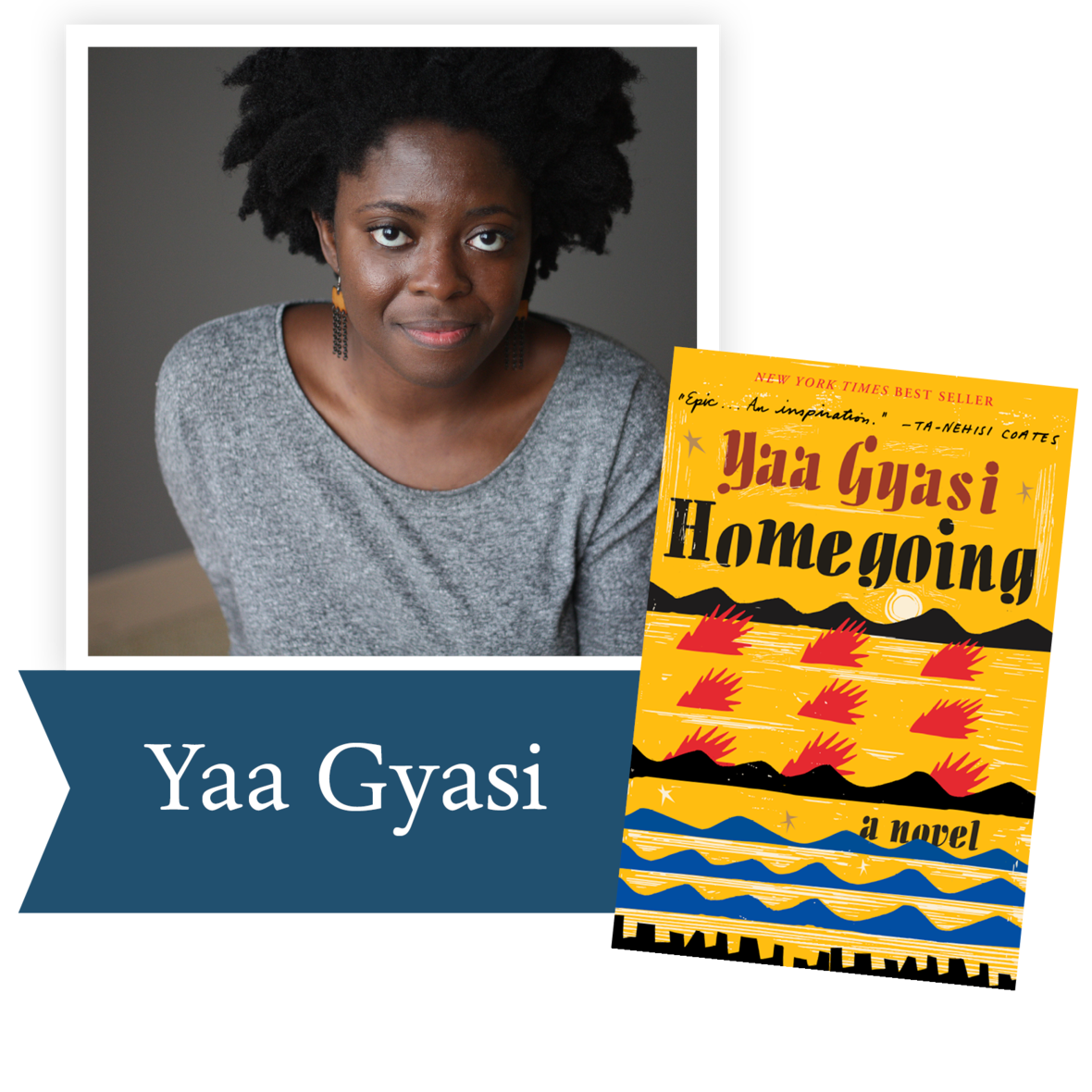2017, Homegoing by Yaa Gyasi (Alfred A. Knopf): A riveting, kaleidoscopic debut novel: a story of race, history, ancestry, love, and time that traces the descendants of two sisters torn apart in eighteenth-century Africa across three hundred years in Ghana and America.

 table div table+table+table+table+table+table+table+table+table+table+table+table+table+table+table+table+table+table div table{width:100%;padding:0}table div table+table+table+table+table+table+table+table+table+table+table+table+table+table+table+table+table+table div table img{width:96.23%;padding:0;float:none}table div table+table+table+table+table+table+table+table+table+table+table+table+table+table+table+table+table+table div table td{width:100%;padding:0 1.88% 18px}/* styles */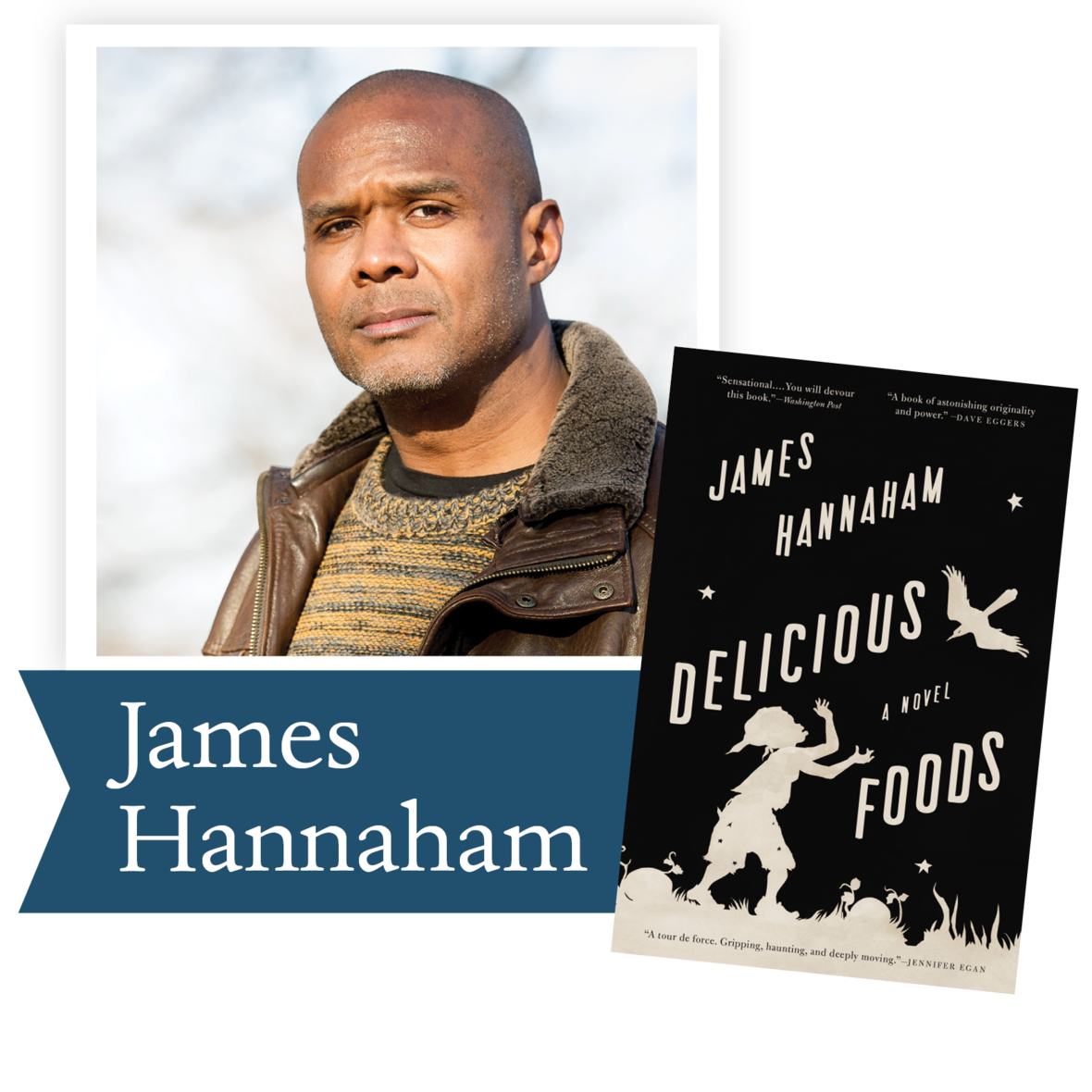2016, Delicious Foods by James Hannaham (Little, Brown and Company): Held captive on a mysterious farm and under the sway of an overpowering addiction, a widow struggles to reunite with her young son in this uniquely American story of freedom, perseverance, and survival. The novel wrestles with timeless questions of love and freedom, forgiveness and redemption, tenacity and the will to survive.

 table div table+table+table+table+table+table+table+table+table+table+table+table+table+table+table+table+table+table+table+table div table{width:100%;padding:0}table div table+table+table+table+table+table+table+table+table+table+table+table+table+table+table+table+table+table+table+table div table img{width:96.23%;padding:0;float:none}table div table+table+table+table+table+table+table+table+table+table+table+table+table+table+table+table+table+table+table+table div table td{width:100%;padding:0 1.88% 18px}/* styles */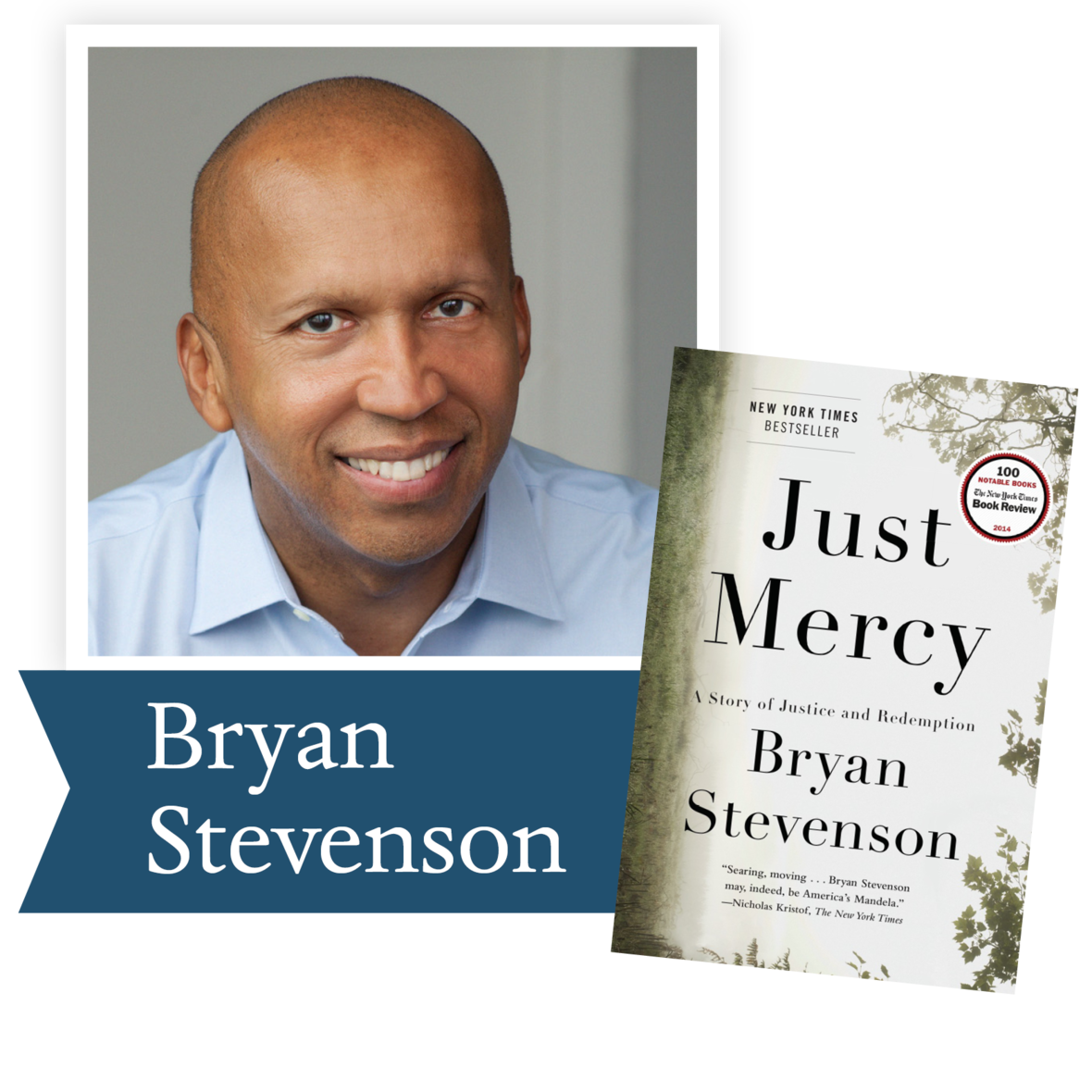2015, Just Mercy by Bryan Stevenson (Spiegel and Grau): From one of the country’s most visionary legal thinkers, social justice advocates, and MacArthur “geniuses,” this is an intimate and unforgettable narrative journey into the broken American criminal justice system, an exquisitely rendered account of a heroic advocate’s fights on behalf of the most powerless people in our society.

 table div table+table+table+table+table+table+table+table+table+table+table+table+table+table+table+table+table+table+table+table+table+table div table{width:100%;padding:0}table div table+table+table+table+table+table+table+table+table+table+table+table+table+table+table+table+table+table+table+table+table+table div table img{width:96.23%;padding:0;float:none}table div table+table+table+table+table+table+table+table+table+table+table+table+table+table+table+table+table+table+table+table+table+table div table td{width:100%;padding:0 1.88% 18px}/* styles */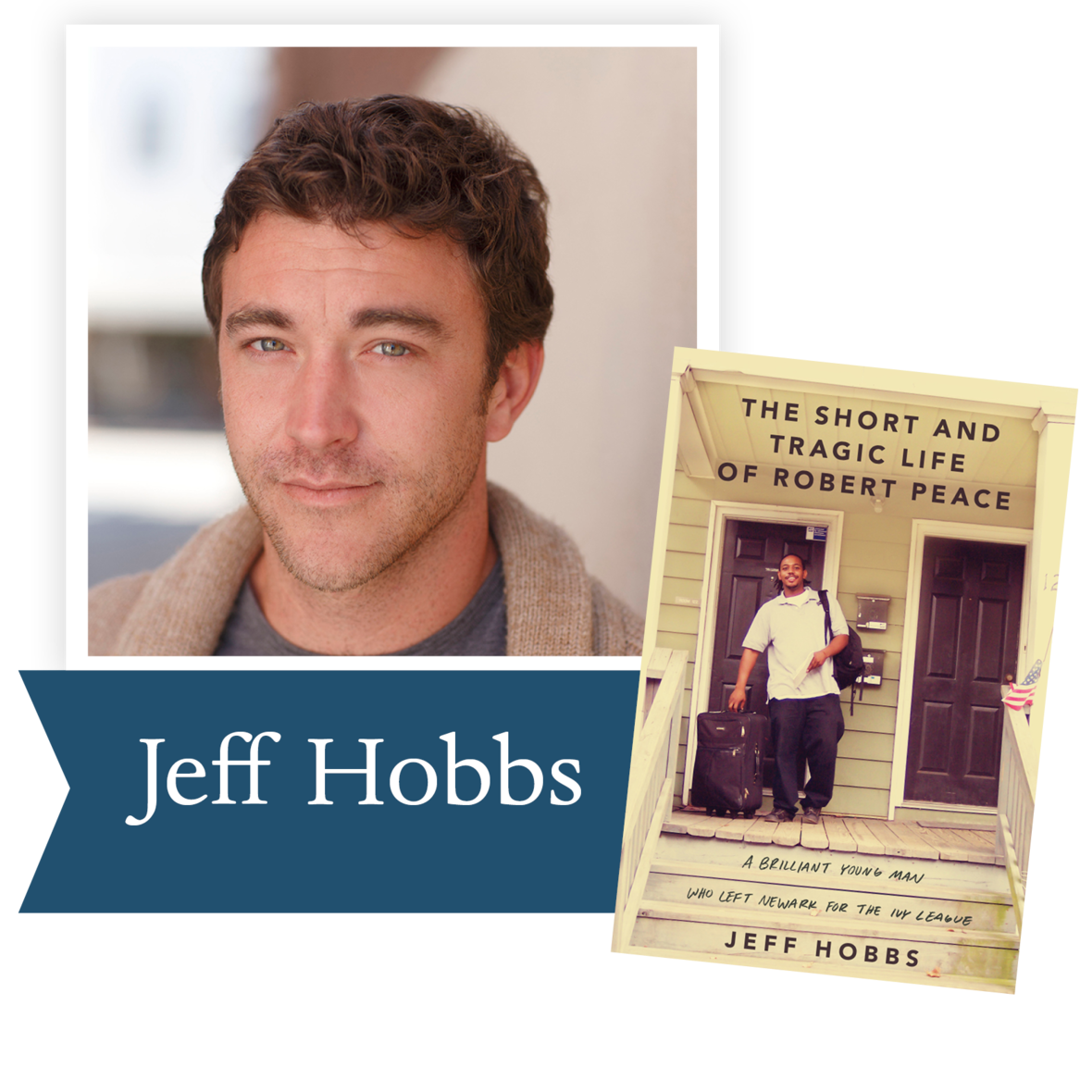2015, The Short and Tragic Life of Robert
Peace by Jeff Hobbs (Scribner): Written by his college roommate, The Short and Tragic Life of Robert Peace is the brilliant, deeply-researched account of the life of Robert DeShaun Peace, a talented young African-American man who left the ghettos of Newark, New Jersey, on a full scholarship to Yale University, but who was tragically murdered in a basement marijuana lab after he graduated.

 table div table+table+table+table+table+table+table+table+table+table+table+table+table+table+table+table+table+table+table+table+table+table+table+table div table{width:100%;padding:0}table div table+table+table+table+table+table+table+table+table+table+table+table+table+table+table+table+table+table+table+table+table+table+table+table div table img{width:96.23%;padding:0;float:none}table div table+table+table+table+table+table+table+table+table+table+table+table+table+table+table+table+table+table+table+table+table+table+table+table div table td{width:100%;padding:0 1.88% 18px}/* styles */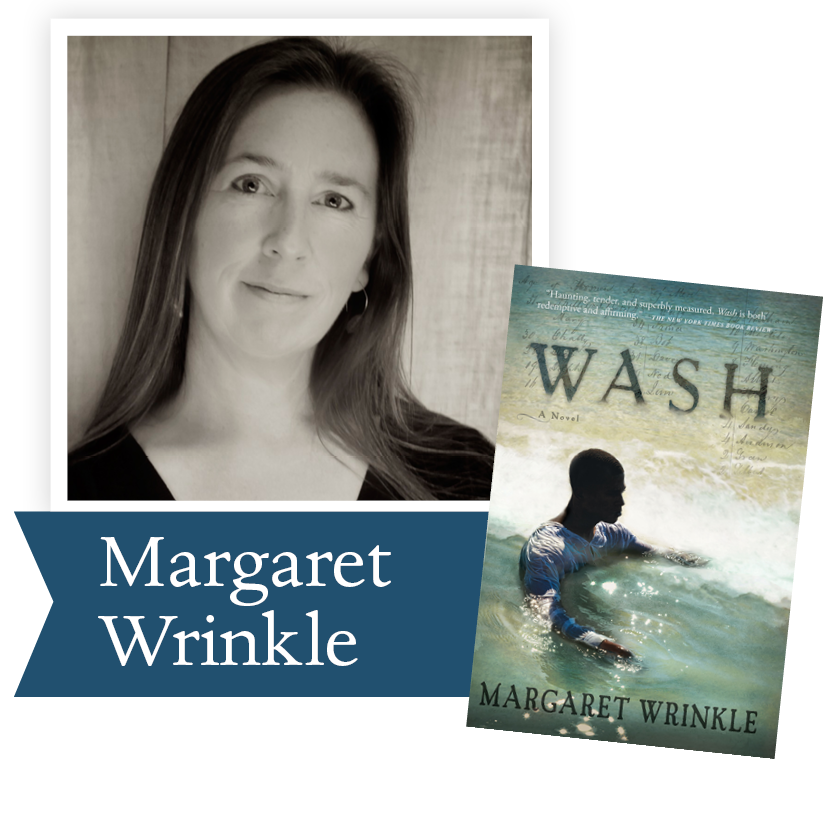2014, Wash by Margaret Wrinkle (Grove Atlantic): Through the character of Wash, a first-generation slave, this haunting first novel explores the often buried history of slave breeding in the early nineteenth century, offering fresh insights into our continuing racial dilemmas.

 table div table+table+table+table+table+table+table+table+table+table+table+table+table+table+table+table+table+table+table+table+table+table+table+table+table+table div table{width:100%;padding:0}table div table+table+table+table+table+table+table+table+table+table+table+table+table+table+table+table+table+table+table+table+table+table+table+table+table+table div table img{width:96.23%;padding:0;float:none}table div table+table+table+table+table+table+table+table+table+table+table+table+table+table+table+table+table+table+table+table+table+table+table+table+table+table div table td{width:100%;padding:0 1.88% 18px}/* styles */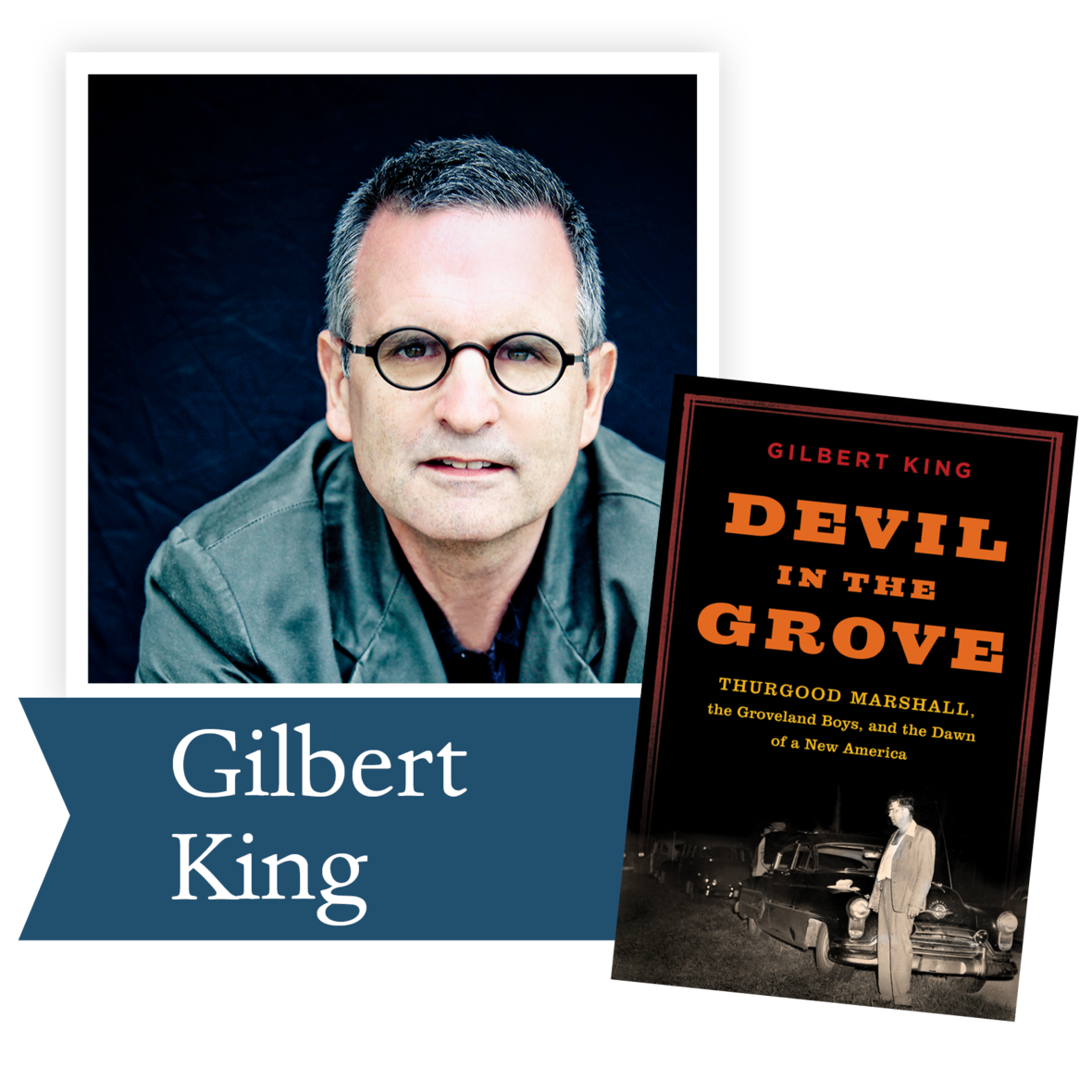2013, Devil in the Grove by Gilbert King
(HarperCollins): A richly detailed chronicle of
four black Florida men who, falsely accused of rape in 1949, were defended by civil rights crusader Thurgood Marshall—later the first African- American Supreme Court justice.

 table div table+table+table+table+table+table+table+table+table+table+table+table+table+table+table+table+table+table+table+table+table+table+table+table+table+table+table+table div table{width:100%;padding:0}table div table+table+table+table+table+table+table+table+table+table+table+table+table+table+table+table+table+table+table+table+table+table+table+table+table+table+table+table div table img{width:96.23%;padding:0;float:none}table div table+table+table+table+table+table+table+table+table+table+table+table+table+table+table+table+table+table+table+table+table+table+table+table+table+table+table+table div table td{width:100%;padding:0 1.88% 18px}/* styles */2011, In the Place of Justice: A Story of Punishment and Deliverance by Wilbert Rideau (Alfred A. Knopf): A death row inmate in Louisiana's Angola penitentiary, at the time the most violent in the nation, finds redemption as a prison journalist in this uplifting memoir.

 table div table+table+table+table+table+table+table+table+table+table+table+table+table+table+table+table+table+table+table+table+table+table+table+table+table+table+table+table+table+table div table{width:100%;padding:0}table div table+table+table+table+table+table+table+table+table+table+table+table+table+table+table+table+table+table+table+table+table+table+table+table+table+table+table+table+table+table div table img{width:96.23%;padding:0;float:none}table div table+table+table+table+table+table+table+table+table+table+table+table+table+table+table+table+table+table+table+table+table+table+table+table+table+table+table+table+table+table div table td{width:100%;padding:0 1.88% 18px}/* styles */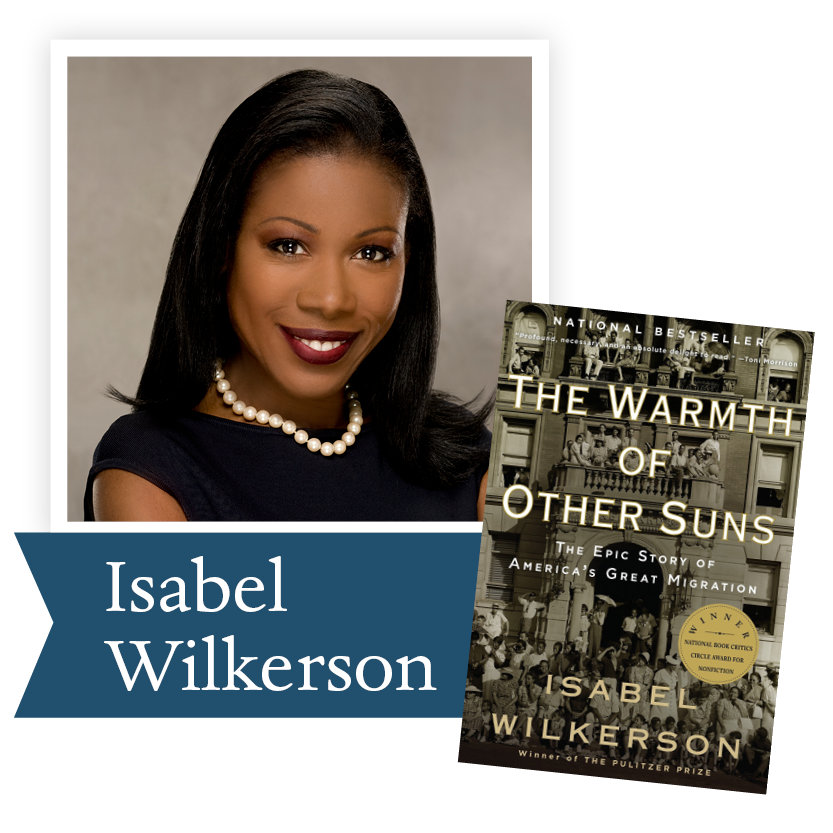2011, The Warmth of Other Suns by Isabel Wilkerson (Random House): Wilkerson tells the stories of three black Americans who fled the South for an uncertain existence in the urban North and West in what became known as the Great Migration of the mid-20th century.

 table div table+table+table+table+table+table+table+table+table+table+table+table+table+table+table+table+table+table+table+table+table+table+table+table+table+table+table+table+table+table+table+table div table{width:100%;padding:0}table div table+table+table+table+table+table+table+table+table+table+table+table+table+table+table+table+table+table+table+table+table+table+table+table+table+table+table+table+table+table+table+table div table img{width:96.23%;padding:0;float:none}table div table+table+table+table+table+table+table+table+table+table+table+table+table+table+table+table+table+table+table+table+table+table+table+table+table+table+table+table+table+table+table+table div table td{width:100%;padding:0 1.88% 18px}/* styles */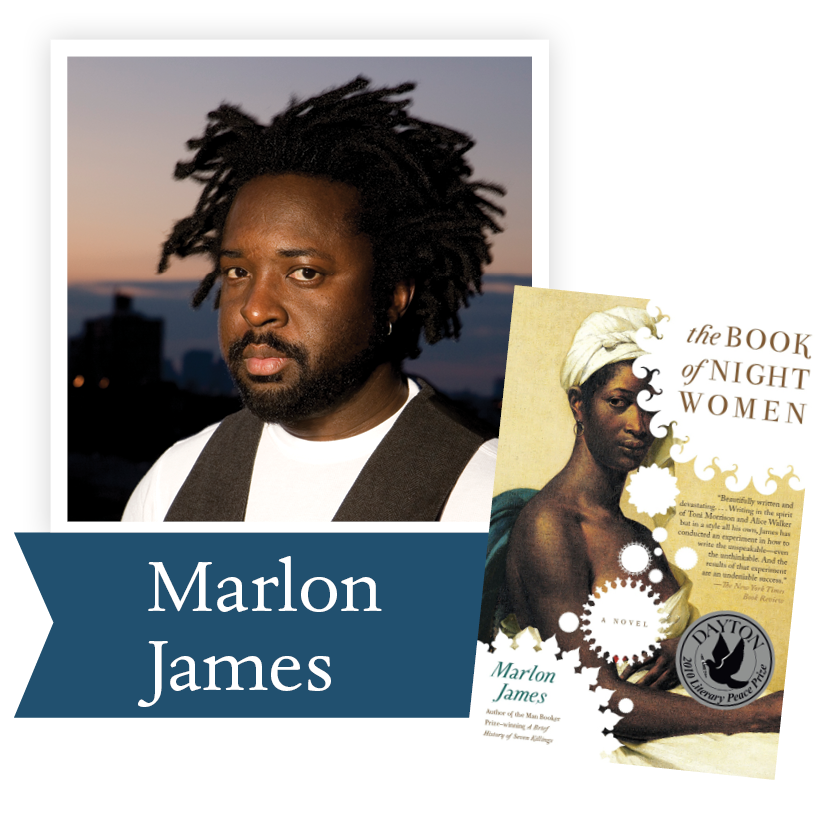2010, The Book of Night Women by Marlon James (Penguin Group; G. P. Putham's Sons/Riverhead Books): Born into slavery on a Jamaican sugar plantation at the end of the eighteenth century, a woman with dark, mysterious powers finds herself at the heart of a slave revolt plotted by the women around her.

 table div table+table+table+table+table+table+table+table+table+table+table+table+table+table+table+table+table+table+table+table+table+table+table+table+table+table+table+table+table+table+table+table+table+table div table{width:100%;padding:0}table div table+table+table+table+table+table+table+table+table+table+table+table+table+table+table+table+table+table+table+table+table+table+table+table+table+table+table+table+table+table+table+table+table+table div table img{width:96.23%;padding:0;float:none}table div table+table+table+table+table+table+table+table+table+table+table+table+table+table+table+table+table+table+table+table+table+table+table+table+table+table+table+table+table+table+table+table+table+table div table td{width:100%;padding:0 1.88% 18px}/* styles */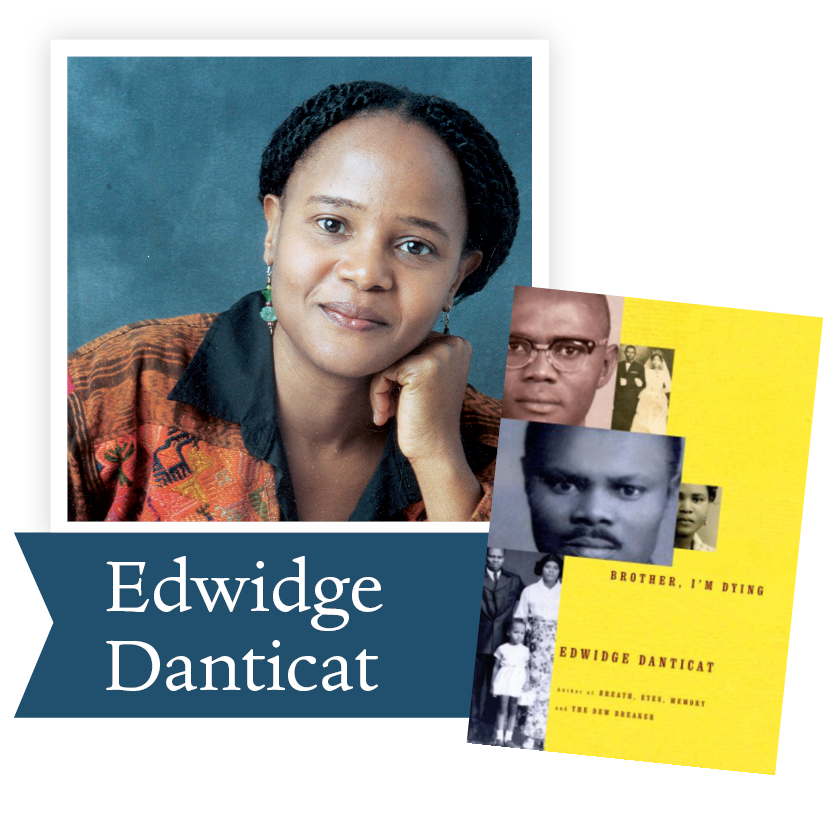2008, Brother, I'm Dying by Edwidge Danticat (Knopf Publishing Group): A moving memoir of Danticat’s Haitian immigrant family’s struggle to stay connected in spite of living apart.

 table div table+table+table+table+table+table+table+table+table+table+table+table+table+table+table+table+table+table+table+table+table+table+table+table+table+table+table+table+table+table+table+table+table+table+table+table div table{width:100%;padding:0}table div table+table+table+table+table+table+table+table+table+table+table+table+table+table+table+table+table+table+table+table+table+table+table+table+table+table+table+table+table+table+table+table+table+table+table+table div table img{width:96.23%;padding:0;float:none}table div table+table+table+table+table+table+table+table+table+table+table+table+table+table+table+table+table+table+table+table+table+table+table+table+table+table+table+table+table+table+table+table+table+table+table+table div table td{width:100%;padding:0 1.88% 18px}/* styles */2008, The Brief Wondrous Life of Oscar Wao by Junot Díaz (Penguin Group): Mixing pop culture and political criticism, this critically acclaimed novel is a warm and humorous account of the American immigrant experience.

 table div table+table+table+table+table+table+table+table+table+table+table+table+table+table+table+table+table+table+table+table+table+table+table+table+table+table+table+table+table+table+table+table+table+table+table+table+table+table div table{width:100%;padding:0}table div table+table+table+table+table+table+table+table+table+table+table+table+table+table+table+table+table+table+table+table+table+table+table+table+table+table+table+table+table+table+table+table+table+table+table+table+table+table div table img{width:96.23%;padding:0;float:none}table div table+table+table+table+table+table+table+table+table+table+table+table+table+table+table+table+table+table+table+table+table+table+table+table+table+table+table+table+table+table+table+table+table+table+table+table+table+table div table td{width:100%;padding:0 1.88% 18px}/* styles */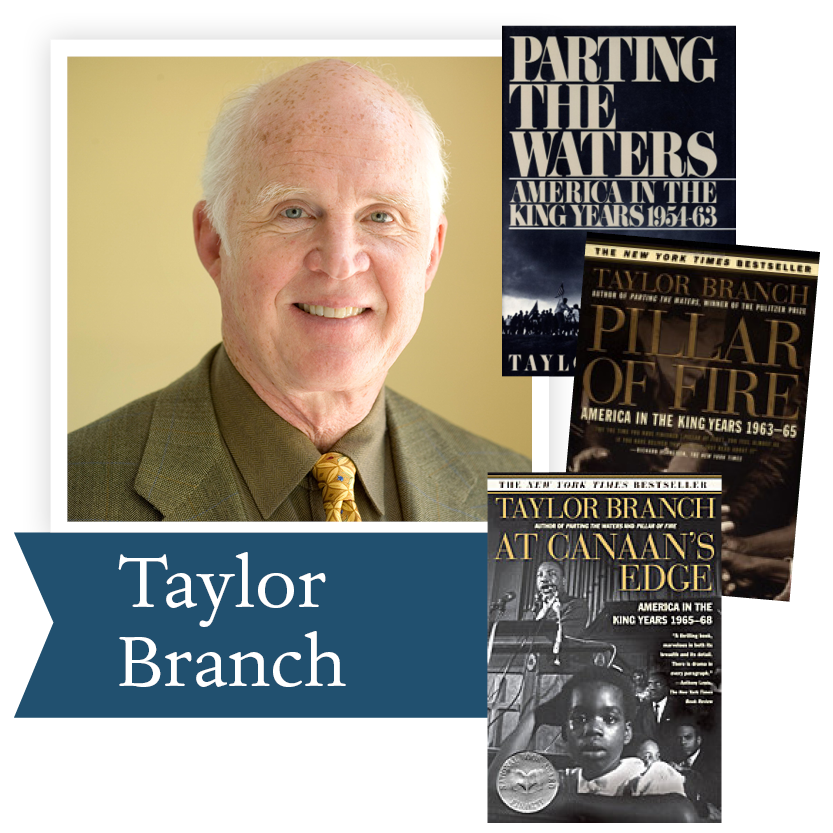2008, In Parting The Waters, Taylor Branch has created an unparalleled epic of America in the midst of change, poised on the threshold of its most explosive era.

In PIllar of Fire, the second volume of his three-part history, Taylor Branch portrays the Civil Rights Movement at its zenith, recounting the climactic struggles as they commanded the national stage.

At Canaan’s Edge concludes America in the King Years, a three-volume history that will endure as a masterpiece of storytelling on American race, violence, and democracy. Pulitzer Prize-winner and bestselling author Taylor Branch makes clear in this magisterial account of the civil rights movement that Martin Luther King, Jr., earned a place next to James Madison and Abraham Lincoln in the pantheon of American history.

 table div table+table+table+table+table+table+table+table+table+table+table+table+table+table+table+table+table+table+table+table+table+table+table+table+table+table+table+table+table+table+table+table+table+table+table+table+table+table+table+table div table{width:100%;padding:0}table div table+table+table+table+table+table+table+table+table+table+table+table+table+table+table+table+table+table+table+table+table+table+table+table+table+table+table+table+table+table+table+table+table+table+table+table+table+table+table+table div table img{width:96.23%;padding:0;float:none}table div table+table+table+table+table+table+table+table+table+table+table+table+table+table+table+table+table+table+table+table+table+table+table+table+table+table+table+table+table+table+table+table+table+table+table+table+table+table+table+table div table td{width:100%;padding:0 1.88% 18px}/* styles */Support the Dayton Literary Peace Prize when you shop with Amazon using this link.